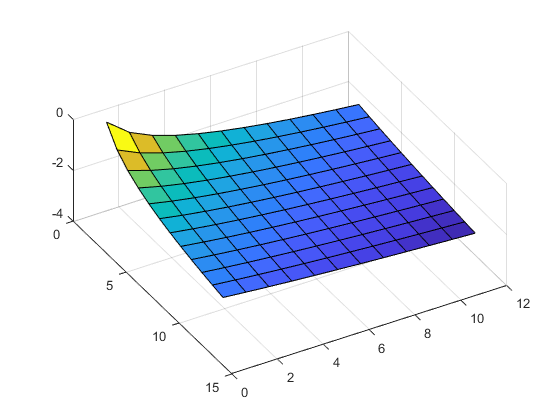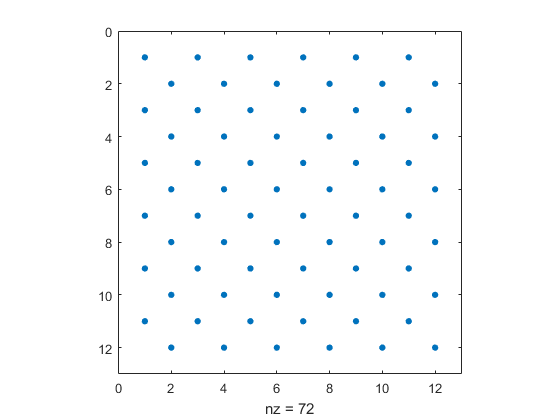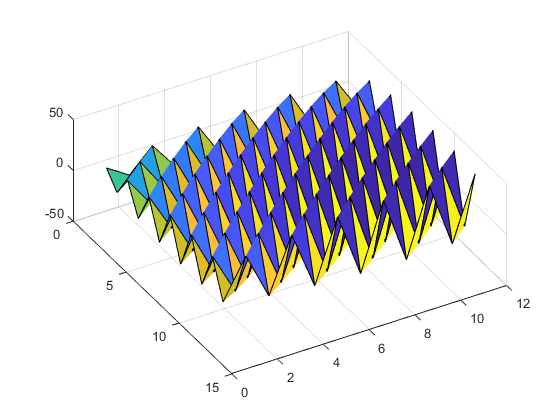# Hilbert Matrices

I first encountered the Hilbert matrix when I was doing individual studies under Professor John Todd at Caltech in 1960. It has been part of my professional life ever since.

### Contents

#### David Hilbert

Around the turn of the 20th century, David Hilbert was the world's most famous mathematician. He introduced the matrix that now bears his name in a paper in 1895. The elements of the matrix, which are reciprocals of consecutive positive integers, are constant along the antidiagonals.

$$h_{i,j} = \frac{1}{i+j-1}, \ \ i,j = 1:n$$

    format rat
H5 = hilb(5)

H5 =
1              1/2            1/3            1/4            1/5
1/2            1/3            1/4            1/5            1/6
1/3            1/4            1/5            1/6            1/7
1/4            1/5            1/6            1/7            1/8
1/5            1/6            1/7            1/8            1/9

    latex(sym(H5));


$$H_5 = \left(\begin{array}{ccccc} 1 & \frac{1}{2} & \frac{1}{3} & \frac{1}{4} & \frac{1}{5}\\ \frac{1}{2} & \frac{1}{3} & \frac{1}{4} & \frac{1}{5} & \frac{1}{6}\\ \frac{1}{3} & \frac{1}{4} & \frac{1}{5} & \frac{1}{6} & \frac{1}{7}\\ \frac{1}{4} & \frac{1}{5} & \frac{1}{6} & \frac{1}{7} & \frac{1}{8}\\ \frac{1}{5} & \frac{1}{6} & \frac{1}{7} & \frac{1}{8} & \frac{1}{9} \end{array}\right)$$

Here's a picture. The continuous surface generated is very smooth.

    H12 = hilb(12);
surf(log(H12))
view(60,60)#### Least squares

Hilbert was interested in this matrix because it comes up in the least squares approximation of a continuous function on the unit interval by polynomials, expressed in the conventional basis as linear combinations of monomials.

$$p(x) = \sum_{j=1}^n c_j x^{j-1}$$

The coefficient matrix for the normal equations has elements

$$\int_0^1 x^{i+j-2} dx \ = \ \frac{1}{i+j-1}$$

#### Properties

A Hilbert matrix has many useful properties.

• Symmetric.
• Positive definite.
• Hankel, $a_{i,j}$ is a function of $i+j$.
• Cauchy, $a_{i,j} = 1/(x_i - y_j)$.
• Nearly singular.

Each column of a Hilbert matrix is nearly a multiple of the other columns. So the columns are nearly linearly dependent and the matrix is close to, but not exactly, singular.

#### hilb

MATLAB has always had functions hilb and invhilb that compute the Hilbert matrix and its inverse. The body of hilb is now only two lines.

J = 1:n;
H = 1./(J'+J-1);


We often cite this as a good example of singleton expansion. A column vector is added to a row vector to produce a matrix, then a scalar is subtracted from that matrix, and finally the reciprocals of the elements produce the result.

#### Inverse

It is possible to express the elements of the inverse of the Hilbert matrix in terms of binomial coefficients. For reasons that I've now forgotten, I always use $T$ for $H^{-1}$.

$$t_{i,j} = (-1)^{i+j} (i+j-1) {{n+i-1} \choose {n-j}} {{n+j-1} \choose {n-i}} {{i+j-2} \choose {i-1}}^2$$

The elements of the inverse Hilbert matrix are integers, but they are large integers. The largest ones are on the diagonal. For order 13, the largest element is

$$(T_{13})_{9,9} \ = \ 100863567447142500$$

This is over $10^{17}$ and is larger than double precision flintmax.

    format longe
flintmax = flintmax

flintmax =
9.007199254740992e+15


So, it is possible to represent the largest elements exactly only if $n \le 12$.

The HELP entry for invhilb includes a sentence inherited from my original Fortran MATLAB.

  The result is exact for  N  less than about 15.

Now that's misleading. It should say

  The result is exact for  N  <= 12.

(I'm filing a bug report.)

#### invhilv

The body of invhilb begins by setting p to the order n. The doubly nested for loops then evaluate the binomial coefficient formula recursively, avoiding unnecessary integer overflow.

    dbtype invhilb 18:28

18    p = n;
19    for i = 1:n
20        r = p*p;
21        H(i,i) = r/(2*i-1);
22        for j = i+1:n
23            r = -((n-j+1)*r*(n+j-1))/(j-1)^2;
24            H(i,j) = r/(i+j-1);
25            H(j,i) = r/(i+j-1);
26        end
27        p = ((n-i)*p*(n+i))/(i^2);
28    end


I first programmed this algorithm in machine language for the Burroughs 205 Datatron at Caltech almost 60 years ago. I was barely out of my teens.

Here's the result for n = 6.

    format short
T6 = invhilb(6)

T6 =
36        -630        3360       -7560        7560       -2772
-630       14700      -88200      211680     -220500       83160
3360      -88200      564480    -1411200     1512000     -582120
-7560      211680    -1411200     3628800    -3969000     1552320
7560     -220500     1512000    -3969000     4410000    -1746360
-2772       83160     -582120     1552320    -1746360      698544


A checkerboard sign pattern with large integers in the inverse cancels the smooth surface of the Hilbert matrix itself.

    T12 = invhilb(12);
spy(T12 > 0)A log scale mitigates the jaggedness.

    surf(sign(T12).*log(abs(T12)))
view(60,60)#### Rookie experiment

Now using MATLAB, I am going to repeat the experiment that I did on the Burroughs 205 when I was still a rookie. I had just written my first program that used Gaussian elimination to invert matrices. I proceeded to test it by inverting Hilbert matrices and comparing the results with the exact inverses. (Today's code uses this utility function that picks out the largest element in a matrix.)

    maxabs = @(X) max(double(abs(X(:))));


Here is n = 10.

    n = 10
H = hilb(n);
X = inv(H);        % Computed inverse
T = invhilb(n);    % Theoretical inverse
E = X - T;         % The error
err = maxabs(E)

n =
10
err =
5.0259e+08


At first I might have said:

Wow! The error is $10^8$. That's a pretty big error. Can I trust my matrix inversion code? What went wrong?

But I knew the elements of the inverse are huge. We should be looking at relative error.

   relerr = maxabs(E)/maxabs(T)

relerr =
1.4439e-04


OK. The relative error is $10^{-4}$. That still seems like a lot.

I knew that the Hilbert matrix is nearly singular. That's why I was using it. John Todd was one of the first people to write about condition numbers. An error estimate that involves nearness to singularity and the floating point accuracy would be expressed today by

   esterr = cond(H)*eps

esterr =
0.0036


That was about all I understood at the time. The roundoff error in the inversion process is magnified by the condition number of the matrix. And, the error I observe is less than the estimate that this simple analysis provides. So my inversion code passes this test.

#### Deeper explanation

I met Jim Wilkinson a few years later and came to realize that there is more to the story. I'm not actually inverting the Hilbert matrix. There are roundoff errors involved in computing H even before it is passed to the inversion routine.

Today, the Symbolic Math Toolbox helps provide a deeper explanation. The 'f' flag on the sym constructor says to convert double precision floating point arguments exactly to their rational representation. Here's how it works in this situation. To save space, I'll print just the first column.

    H = hilb(n);
F = sym(H,'f');
F(:,1)

ans =
1
1/2
6004799503160661/18014398509481984
1/4
3602879701896397/18014398509481984
6004799503160661/36028797018963968
2573485501354569/18014398509481984
1/8
2001599834386887/18014398509481984
3602879701896397/36028797018963968


The elements of H that are not exact binary fractions become ratios of large integers. The denominators are powers of two; in this case $2^{54}$ and $2^{55}$. The numerators are these denominators divided by $3$, $5$, etc. and then rounded to the nearest integer. The elements of F are as close to the exact elements of a Hilbert matrix as we can get in binary floating point.

Let's invert F, using the exact rational arithmetic provided by the Symbolic Toolbox. (I couldn't do this in 1960.)

    S = inv(F);


We now have three inverse Hilbert matrices, X, S, and T.

• X is the approximate inverse computed with floating point arithmetic by the routine I was testing years ago, or by MATLAB inv function today.
• S is the exact inverse of the floating point matrix that was actually passed to the inversion routine.
• T is the exact Hilbert inverse, obtained from the binomial coefficient formula.

Let's print the first columns alongside each other.

   fprintf('%12s %16s %15s\n','X','S','T')
fprintf('%16.4f %16.4f %12.0f\n',[X(:,1) S(:,1) T(:,1)]')

           X                S               T
99.9961          99.9976          100
-4949.6667       -4949.7926        -4950
79192.8929       79195.5727        79200
-600535.3362     -600559.6914      -600600
2522211.3665     2522327.5182      2522520
-6305451.1288    -6305770.4041     -6306300
9608206.6797     9608730.4926      9609600
-8750253.0592    -8750759.2546     -8751600
4375092.6697     4375358.4162      4375800
-923624.4113     -923682.8529      -923780


It looks like X is closer to S than S is to T. Let's confirm by computing two relative errors, the difference between X and S, and the difference between S and T.

    format shorte
relerr(1) = maxabs(X - S)/maxabs(T);
relerr(2) = maxabs(S - T)/maxabs(T)
relerrtotal = sum(relerr)

relerr =
5.4143e-05   9.0252e-05
relerrtotal =
1.4439e-04


The error in the computed inverse comes from two sources -- generating the matrix in the first place and then computing the inverse. The first of these is actually larger than the second, although the two are comparable.

Published with MATLAB® R2017a

|